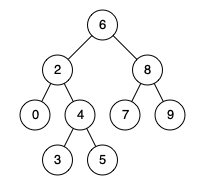# 若只如初见

0%

## 问题描述

Given a binary search tree (BST), find the lowest common ancestor (LCA) of two given nodes in the BST.

According to the definition of LCA on Wikipedia: “The lowest common ancestor is defined between two nodes p and q as the lowest node in T that has both p and q as descendants (where we allow a node to be a descendant of itself).”

Example 1:Example 2:

Example 3:

Constraints:

• The number of nodes in the tree is in the range [2, 105].
• -109 <= Node.val <= 109
• All Node.val are unique.
• p != q
• p and q will exist in the BST.

## 解题思路

• 二叉搜索树，若是p，q比当前根结点小，则父节点必在左子树中，反之，则在右子树中。

• 代码如下：

• JavaScript

• 时间复杂度：O(n)
• 空间复杂度：O(1)